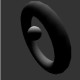by ruslany

Compositor Pro v3 is able to reproduce Chladni patterns in 3-dimensional field. First, I will say you a little about the approach named in Compositor – the bottom-up approach paper. The one period of FM waveform is an estimation between the moving plate and the static one as in experiment named in Air-induced inverse Chladni patterns paper by Henk Jan van Gerner et al. The reason I write this is to aim the possibility of creating such patterns using the Compositor Pro output not only as OpenGL 3d model but as a physical reality experiment. For this it is needed the sufficient amount of sound amplification not found at my studio or any laboratory. The amplification should be considered as an amount found on modern concert places such as:The image below shows the difference between two named vectors, which estimate the path moleculas taken between static and moving plate.My image shows an exactly the same process but only in 3d field:The process on the gif image is called spherical interaction by a method implied. The use of algorithm function is as follows:

1. Use Bessel function values to estimate each harmonic amplitude as x axis
2. Use Phase as y axis
3. Use Frequency as z axis
4. Convert those values into spherical coordinates

This way I got a vector in three dimensional spherical field. If we will represent the seen Universe as a sphere hence this could be a coordinates for space-time travel.